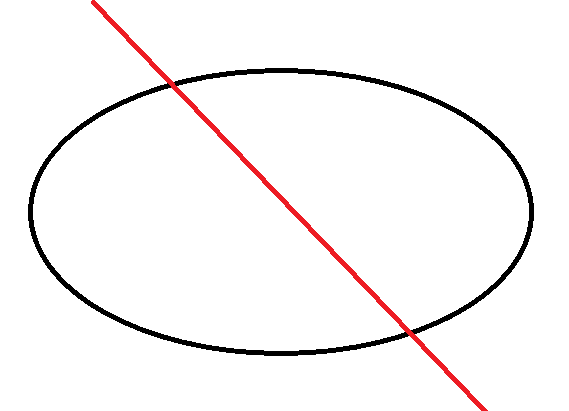# How to flip an image about a line defined by me

I have a 3D volume data and I want to flip the image (not with the central vertical line) with a plane that is predefined. Is there any work done in this direction in itk or sitk?Hello,

This can be done in C++ ITK, Python ITK, or SimpleITK with the ResampleImageFilter and the proper transform. You will want to set the filter’s reference image to the same image as the input, so the output geometry is the same as the input.

By “flip” I’m assuming you mean mirror. In 3d the problem will only be well defined if you are flipping on a plane ( not a line ). You will have to construct the transform matrix by composing or multiplying 3 affine matrices:

T = R * F * R^{-1},

where R is a rotation matrix to align the plan with an axis. F is a flip or mirror on the axis and R^{-1} is the inverse of the rotation.

Hopefully that is enough to get you started in the right direction with your problem to discover the right components in ITK and appropriate math to consult.

1 Like

Thanks for the information. I will try in this direction.
Can’t i use the code below for my problem? If yes, how to define the axis? https://itk.org/ITKExamples/src/Filtering/ImageGrid/FlipAnImageOverSpecifiedAxes/Documentation.html

That example can only flip across X, Y, or Z axes, not arbitrary ones.

You can determine the correct mirroring transformation (that you can use as input for image resampling) by landmark-based transform initializer. You need to specify a two lists of corresponding points as inputs - for example first list can contain 5 anatomical landmarks on the left side and the second list contains the corresponding points on the right side.

1 Like